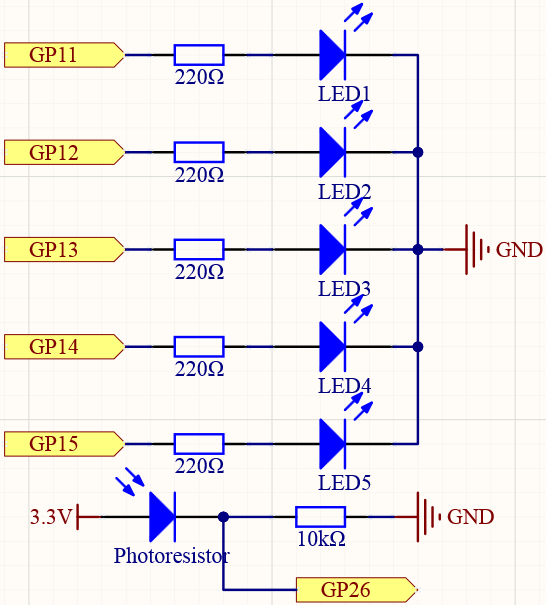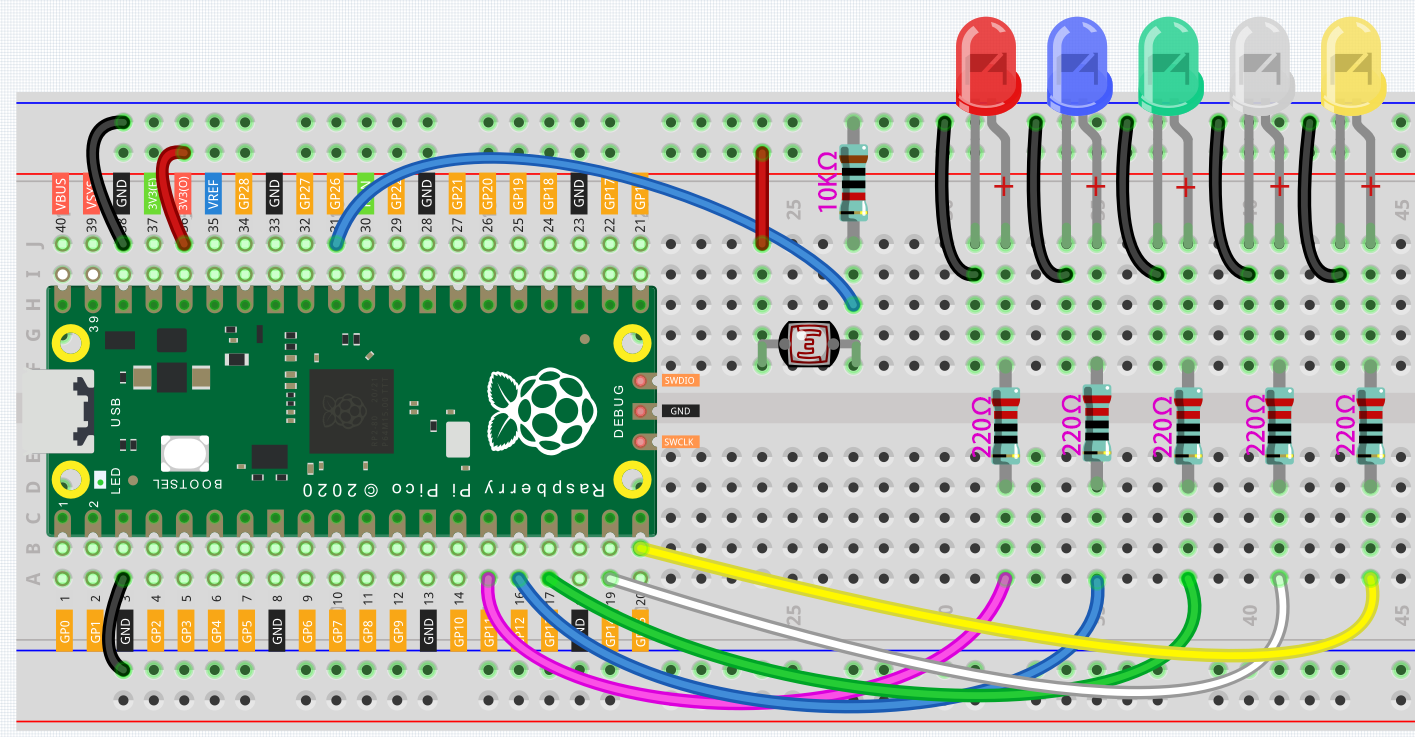# Measure Light Intensity¶

A photoresistor or photocell is a light-controlled variable resistor. The resistance of a photo resistor decreases with increasing incident light intensity; in other words, it exhibits photo conductivity.

Therefore, we can use a photoresistor to measure light intensity, and then show it through 5 LEDs.

## Schematic¶## Wiring¶

In this experiment, we will use 5 LEDs to show the light intensity. The higher the light intensity is, the more LEDs will light up, vice versa.## How it works?¶

```const int ledPins[] = {11, 12, 13, 14, 15};
const int photocellPin = A0;  //photoresistor attach to A0
int sensorValue = 0;        // value read from the sensor
int Level = 0;           // sensor value converted into LED 'bars'
```

First of all, there are still various initialization definitions, setting pins and setting initial values ​​of variables.

In order to quickly set the input/output status and HIGH/LOW for the 5 LEDs in the following code, here we use the array `ledPin[]` to define the 5 LEDs connected to the corresponding pins of the Pico.

The element number of the array usually starts from 0. For example, `ledPin` refers to GPIO11, and `ledPin` refers to GPIO15.

```void setup()
{
Serial.begin(9600);  // start serial port at 9600 bps:
for (int i = 0; i < 5; i++)
{
pinMode(ledPins[i], OUTPUT);// make all the LED pins outputs
}
}
```

In `setup()`，using the `for()` statement set the 5 pins to OUTPUT. The variable `i` is added from 0 to 5, and the `pinMode()` function sets pin11 to pin15 to OUTPUT in turn.

```sensorValue = analogRead(photocellPin); //read the value of A0
Level = map(sensorValue, 0, 1023, 0, 5);  // map to the number of LEDs
Serial.println(Level);
delay(10);
```

In `loop()`, read the analog value of the photocellPin(A0) and store to the variable sensorValue.

The `map()` function is used to map 0-1023 to 0-5. It means that the value range of the photoresistor (0-1023) is equally divided into 5 levels, 0-204.8 belongs to Level 0, 204.9-409.6 belongs to Level 1, and 819.2-1023 belong to Level 4. If the value of variable `sensorValue` is 300 at this time, then `Level` is equal to 1.

```for (int i = 0; i < 5; i++)
{
if (i <= Level ) //When i is smaller than Level, run the following code.
{
digitalWrite(ledPins[i], HIGH);     // turn on LEDs less than the level
}
else
{
digitalWrite(ledPins[i], LOW);     // turn off LEDs higher than level
}
}
```

Now we need to find a way to display the brightness level at this time with LEDs.

The `for()` statement is used here to perform loop detection in the `ledPin[]` array. If the element bit in the array is less than the value of `Level`, the corresponding GPIO is set to high level, that is, the corresponding LED is lit. If `Level` is equal to 1, turn on the LEDs on GPIO11 and GPIO12.